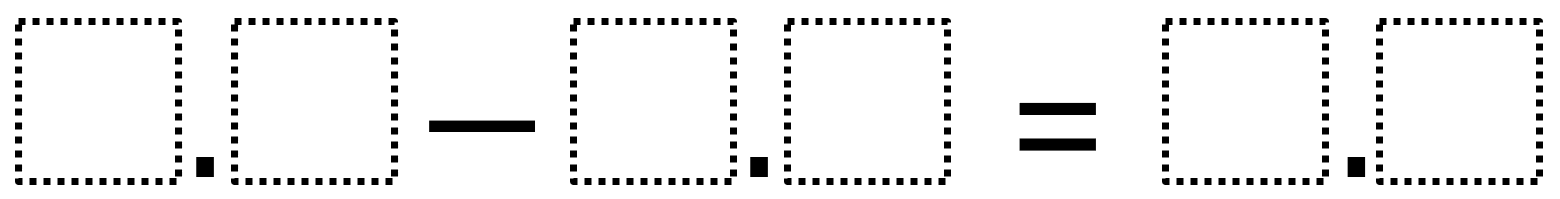Home > Grade 5 > Decimal Subtraction

# Decimal Subtraction

Directions: Use the digits 1 to 9, at most one time each, to make two true statements.### Hint

How do you place digits so the difference is less than ten?

### Answer

There are many answers including:
6.8 – 4.1 = 2.7
9.1 – 5.7 = 3.8

Source: Owen Kaplinsky

## Multiplying Decimals to Make a Whole Number Product

Directions: Using the digits 1 to 9 at most one time each, fill in the …

### One comment

1.Mrs. Elliott's 5th Graders

5.9-1.2=4.7
7.9-8.3=1.6
3.9-1.5=2.4
8.7-2.3=6.4
9.8-6.1=2.7
8.1-4.6=3.5
9.5-8.3=1.2
9.7-8.5=1.2